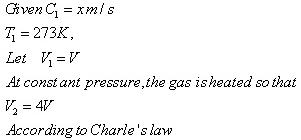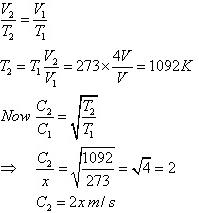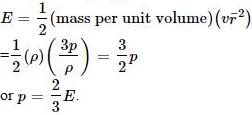Courses

# Test: Kinetic Theory Of Gases

## 10 Questions MCQ Test Physics Class 11 | Test: Kinetic Theory Of Gases

Description
This mock test of Test: Kinetic Theory Of Gases for Class 11 helps you for every Class 11 entrance exam. This contains 10 Multiple Choice Questions for Class 11 Test: Kinetic Theory Of Gases (mcq) to study with solutions a complete question bank. The solved questions answers in this Test: Kinetic Theory Of Gases quiz give you a good mix of easy questions and tough questions. Class 11 students definitely take this Test: Kinetic Theory Of Gases exercise for a better result in the exam. You can find other Test: Kinetic Theory Of Gases extra questions, long questions & short questions for Class 11 on EduRev as well by searching above.
QUESTION: 1

### At absolute zero temperature may be defined as that temperature at which

Solution:

At 0K temperature we know that there is no molecular motion, that is the KE of the particles gets 0. Thus we can say the combined KE of a gaseous system is zero, but as there combined mass cant be zero thus the combined of the square of velocities of the particles is zero, which means that the root mean square velocity of the gas is zero.

QUESTION: 2

Solution:
QUESTION: 3

### If the r.m.s speed of oxygen at NTP is x m/s. If the gas is heated at constant pressure till its volume is four fold, what will be its final temperature and r.m.s speed?

Solution:QUESTION: 4

The perfect gas equation is PV = nRT where n is the

Solution:

n is the number of moles.

QUESTION: 5

In a mixture of ideal gases at a fixed temperature the heavier molecule has the lower average speed. This is easiest to conclude from the equation

Solution:

In option A, v is inversely proportional to mass. Hence, the condition of the question is seen accurately in that option.

QUESTION: 6

According to kinetic theory of gases, 0K is that temperature at which for an ideal gas

Solution:

According to kinetic theory of gases, 0K is that temperature at which for an ideal gas the internal energy is zero because at 0K nearly all molecular motion stops.

QUESTION: 7

The kinetic energy of one mole of an ideal gas is E=3/2 RT. Then Cρ will be

Solution:

We know that,
Cp=cv+R
E=3Rt/2
also,
E=Cv
cp=R+3RT/2
=5/2 RT
=2.5R

QUESTION: 8

Value of gas constant, R for one mole of a gas is independent of the

Solution:

We know that PV=nRT also PM=dRT
So in the equation The value of R depends on P , V , n , T , d , M
except atomicity
so the ans is A

QUESTION: 9

The pressure exerted by an ideal gas is numerically equal to _________ of the mean kinetic energy of translation per unit volume of the gas.

Solution:

Translational (KE) per unit volumeQUESTION: 10

The average kinetic energy of a molecule in an ideal gas is

Solution:

KE = f/2 KT